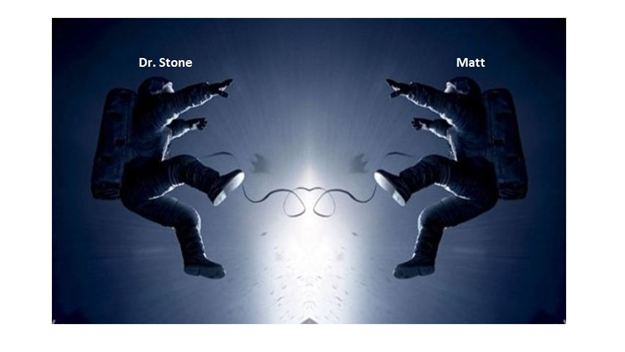# Dr. Stone and Matt meet in spaceDr. Stone and Matt are the two astronauts in the movie "Gravity". Suppose that they are in a gravity-free space pushing each other out as above. If Matt is more massive than Dr. stone, which of the following statements is $\underline{\text{NOT}}$ correct?

a) The magnitude of the impulse is the same for both Dr. Stone and Matt.

b) The sum of the magnitudes of the change in momentum for the two persons is zero.

c) The magnitude of the velocity of Matt after the push is larger than that of Dr. Stone.

×